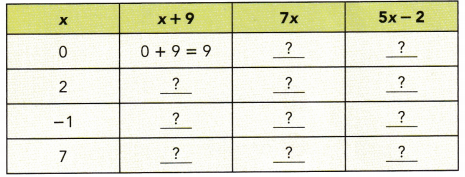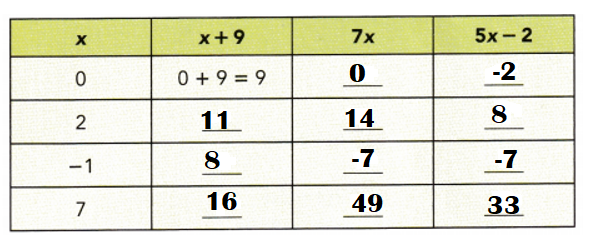# Math in Focus Grade 7 Chapter 3 Answer Key Algebraic Expressions

Go through the Math in Focus Grade 7 Workbook Answer Key Chapter 3 Algebraic Expressions to finish your assignments.

## Math in Focus Grade 7 Course 2 A Chapter 3 Answer Key Algebraic Expressions

### Math in Focus Grade 7 Chapter 3 Quick Check Answer Key

Consider the algebraic expression 3x + 4. State the following.

Question 1.
How many terms are there?
Two terms are there.

Explanation:
Algebraic expression : 3x + 4

Question 2.
State the coefficient of the algebraic term.
3 is coefficient of the algebraic term.

Explanation:
A coefficient is an integer that is written along with a variable or it is multiplied by the variable. In other words, a coefficient is the numerical factor of a term containing constant and variables.
In the term 3x + 4, 3 is the coefficient.

Question 3.
What is the constant term?
4 is the constant term in 3x + 4.

Explanation:
A constant term is a term in an algebraic expression that does not contain any variables and therefore is constant.

Question 4.
Write the operation symbol.
Addition (+) is the operation symbol in 3x + 4.

Explanation:
The symbol indicating a math operation is an operator symbol.

Question 5.
Complete the table.Explanation:
When x = 0:
7x = 7 × 0 = 0.
5x – 2 = 5(0) – 2 = 0 – 2 = – 2.

When x = 2:
x + 9 = 2 + 9 = 11.
7x = 7 × 2 = 14.
5x – 2 = 5(2) – 2 = 10 – 2 = 8.

When x = -1:
x + 9 = -1 + 9 = 8.
7x = 7 × -1 = -7.
5x – 2 = 5(-1) – 2 = -5 – 2 = -7.

When x = 7:
x + 9 = 7 + 9 = 16.
7x = 7 × 7 = 49.
5x – 2 = 5(7) – 2 = 35 – 2 = 33.

State whether each expression can be simplified. Explain your reasoning.
Question 6.
2k – 3 + k
2k – 3 + k = 3(k – 1).

Explanation:
2k – 3 + k
= 3k – 3
= 3(k – 1)

Question 7.
7x + 3 – 3y
7x + 3 – 3y = 7x + 3(1 – y).

Explanation:
7x + 3 – 3y
= 7x + 3(1 – 1y)
= 7x + 3(1 – y)

Question 8.
6u + 5w – 1
6u + 5w – 1 cannot be simplified further as there is no common terms.

Explanation:
6u + 5w – 1
= 6u + 5w – 1.

Question 9.
4g – 3g – g
4g – 3g – g = 0.

Explanation:
4g – 3g – g
= g(4 – 3 – 1)
= g(1 – 1)
= g (0)
= 0.

Simplify each expression.
Question 10.
4t + 1 + 6
4t + 1 + 6 = 4t + 7.

Explanation:
4t + 1 + 6
= 4t + 7.

Question 11.
5p – 5p
5p – 5p = 0.

Explanation:
5p – 5p
= 5p(1 – 1)
= 5p(0)
= 0.

Question 12.
4y + 5y + 3
4y + 5y + 3 = 7y + 3.

Explanation:
4y + 5y + 3
= y(4 + 3) + 3
= y(7) + 3
= 7y + 3.

Question 13.
4m – 3m – 3
4m – 3m – 3 = m – 3.

Explanation:
4m – 3m – 3
= m(4 – 3) – 3
= m(1) – 3
= m – 3.

Expand each expression.
Question 14.
4(h + 2)
4(h + 2) = 4h + 8.

Explanation:
4(h + 2)
= 4h + 8.

Question 15.
5(4 + 5c)
5(4 + 5c) = 20 + 25c.

Explanation:
5(4 + 5c)
= 20 + 25c.

Question 16.
3(4x – 11)
3(4x – 11) = 12x – 33.

Explanation:
3(4x – 11)
= 12x – 33.

Question 17.
7(3 – 5p)
7(3 – 5p) = 21 – 35p.

Explanation:
7(3 – 5p)
= 21 – 35p.

Factor each expression.
Question 18.
6m + 3
6m + 3 = 3(2m + 1).

Explanation:
6m + 3
= 3(2m + 1)

Question 19.
4v + 14
4v + 14 = 2(2v + 7).

Explanation:
4v + 14
= 2(2v + 7)

Question 20.
10p – 2
10p – 2 = 2(5p – 1).

Explanation:
10p – 2
= 2(5p – 1)

Question 21.
6 – 18c
6 – 18c = 6(1 – 3c).

Explanation:
6 – 18c
= 6(1 – 3c).

Choose an equivalent expression.
Question 22.
6y – 3 is equivalent to
a) 3(3y – 1)
b) 3(2y – 1)
c) 3(2y – 3)
d) 6(3y – 1)
6y – 3 is equivalent to 3(2y – 1).
b) 3(2y – 1).

Explanation:
a. 3(3y – 1) = 9y – 3.
b. 3(2y – 1) = 6y – 3.
c. 3(2y – 3) = 6y – 9.
d. 6(3y – 1) = 18y – 6.

x is an unknown number. Write an expression for each of the following.
Question 23.
7 more than the number
Expression = x + 7.

Explanation:
x is an unknown number.
7 more than the number
=> x + 7.

Question 24.
Product of 8 and the number
Expression = 8x.

Explanation:
Product of 8 and the number.
=> 8 × x= 8x.

Question 25.
5 less than twice the number
Expression = 2x – 5.

Explanation:
5 less than twice the number.
=> 2x – 5.

Question 26.
3 more than half the number